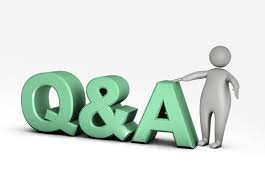# SCC Education

## Matter In Our Surroundings mcqSubject – Science
Matter In Our Surroundings
1. Camphor is purified by the process of –
a. evaporation                  b. distillation                                    c. sublimation                                  d. filtration
2. LPG is used in the kitchens in our homes in the-
a. Solid form                      b. compressed form                      c. gaseous form                               d. none.
3. Which of the following is not fluid –
a. water,                             b. air,                                                   c. sand,                                                d. sharbat?
4. On Kelvin scale 0oC is equal to –
a. 273 K                              b. -273 K                                             c. 0 K                                                    d. 100 K
5. SI unit of temperature is –
a. Kelvin                             b. Celsius                                            c. Fahrenheit                                    d. Pascal
6. Dry ice means –
a. solid SO2                                     b. solid water                                   c. solid CO2                                                             d. solid CO
7. Condensation is a process which involves conversion of –
a. solid into liquid           b. liquid into vapour                     c. vapour into liquid                     d. liquid into solid
8. During evaporation, the particles of liquid evaporate from –
a. The bulk of liquid       b. surface only                                 c. the surface and bulk of liquid                               d. none of them.
9. Which of the following will not sublime –
a. Camphor                        b. ammonium chloride                                                c. bromine                                          d. iodine?
10. A state of matter which has a definite shape and fixed volume –
a. Solid                                 b. liquid                                                              c. gas                                                   d. none of these.

11. A state of matter which does not have a definite shape but has definite volume –
a. Solid                                 b. liquid                                                               c. gas                                                   d. none of these.
12. A state of matter which neither has neither definite shape nor definite volume –
a. Solid                                 b. liquid                                                              c. gas                                                   d. none of these.
13. The states of matter can be changed by changing –
a. Temperature                                b. pressure                                                         c. both (a) and (b)                         d. none of these.
14. The rate of evaporation does not depend on –
a. Temperature                                b. humidity                                                        c. pressure                                          d. speed of wind.
15. Which of the following is accompanied by cooling –
a. Vaporization                                b. evaporation                                                 c. condensation                                              d. none of these.
16. A bulk phenomenon in which particles from whole of the liquid change into vapour state is called -

a. Boiling                            b. cooling                                                           c. melting                                           d. none of these.
17. The amount of heat energy required to change 1 kg of solid into liquid at its melting point is called –

a. Melting point                               b. latent heat of fusion                                                 c. boiling point                 d. latent heat of vaporization.
18. The amount of heat energy required to change 1 kg of liquid into solidat its boiling point is called –
a. Melting point              b. latent heat of fusion                                  c. boiling point                                d. latent heat of vaporization.

19. The density of water is _________ than that of ice.
a. More                               b. less                                   c. equal to                                          d. none.
20. The temperature at which the solid melts to become a liquid at the atmospheric pressure is called –
a. Boiling point                                b. melting point              c. freezing point                              d. none of these.
21. The temperature at which the vapour pressure of the liquid becomes equal to the atmospheric pressure is called –
a. Boiling point                                b. melting point              c. freezing point                              d. none of these.
22. Which is not the characteristic of matter –
a. particles of a matter are continuously moving, b. particles of matter move faster on increasing temperature,
c. particles of matter intermix with each other on their own, d. Particles of all maters have same kinetic energy.
23. The compressibility of which state of matter is high –
a. Solid                                 b. liquid                              c. gas                                                   d. all of these.
24. Which of the following is the simplest form of matter –
a. Element                          b. mixture                          c. compound                                    d. none of these.
25. Which of them is most energetic –
a. Solids                               b. liquids                            c. gases                                               d. all of them.
26. Which of the following is correct for something to be called matter -
a. It should occupy spac              e              b. It should have mass                  c. Both (a) and (b)                         d. None of these.
27. Which of the following cannot be considered as matter –
a. Mixture                                           b. affection                                        c. gas                                                   d. liquid.
28. Which of the following is correct about solid carbon dioxide –
a. It is used in theatres to give special effects.                                    b. It is extremely cold substance.
c. It is used to keep freeze food and to keep ice-cream cold.       d. All of them.
e. Noneof them.
29. Which of the following statements is not correct –
a. The density of ice is less than the density of water.
b. To convert a temperature on the Kelvin scale to Celsius scale, subtract 273 from the given temperature.
c. To convert a temperature on the Celsius scale to Kelvinscale, add 273 to the given temperature.
d. Vaporisation of a liquid causes cooling.
30. When a substance is directly changed form solid state to gas on heating or from gas to solid on cooling, this process is called –
a. Evaporation                 b. condensation                                              c. sublimation                                  d. vaporization